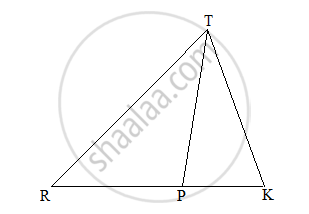# In the following figure RP: PK= 3:2, then find the value of A(ΔTRP):A(ΔTPK). - Geometry

Sum

In the following figure RP: PK= 3:2, then find the value of A(ΔTRP):A(ΔTPK).#### Solution

Ratio of the areas of two triangles with common or equal heights is equal to the ratio of their corresponding bases.

(A(triangleTRP))/(A(triangleTPK))="RP"/"PK"=3/2

Concept: Properties of Ratios of Areas of Two Triangles
Is there an error in this question or solution?